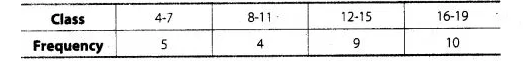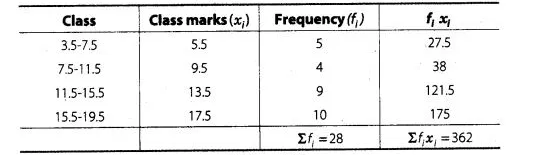# Calculate the mean of the following data

Question:

Calculate the mean of the following dataSolution:

Since, given data is not continuous, so we subtract 0.5 from the lower limit and add 0.5 in the upper limit of each class.

Now, we first find the class mark xi, of each class and then proceed as followsTherefore, $\bar{x}($ mean $)=\frac{\Sigma f_{i} x_{i}}{\Sigma_{j}}=\frac{362}{28}=12.93$

Hence, mean of the given data is $12.93$.